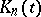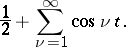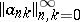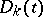# Kernel of a summation method

A function(depending on a parameter) the values of which are the averages of the given method of summation applied to the series(1)

The kernel of a summation method gives an integral representation of the averages of the method in the summation of Fourier series. If the summation method is defined by a transformation of sequences into sequences using a matrix, then the kernel of this method is the functionwhereare the partial sums of the series (1):(2)

In this case the averages of the Fourier series for a-periodic functioncan be expressed in terms ofand the kernel by the formulaIn particular, the kernel of the method of arithmetical averages (cf. Arithmetical averages, summation method of) has the formand is called the Fejér kernel. The kernel of the Abel summation method is given byand is called the Poisson kernel. The functionin (2) is called the Dirichlet kernel.

The functionwhose values are the averages of a summation method applied to the seriesis called the conjugate kernel of the summation method.